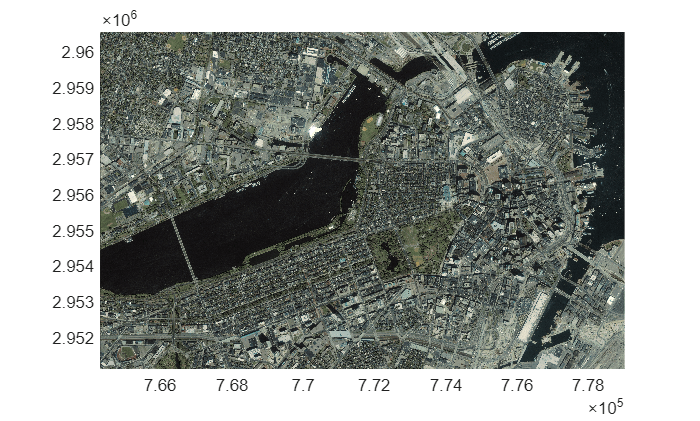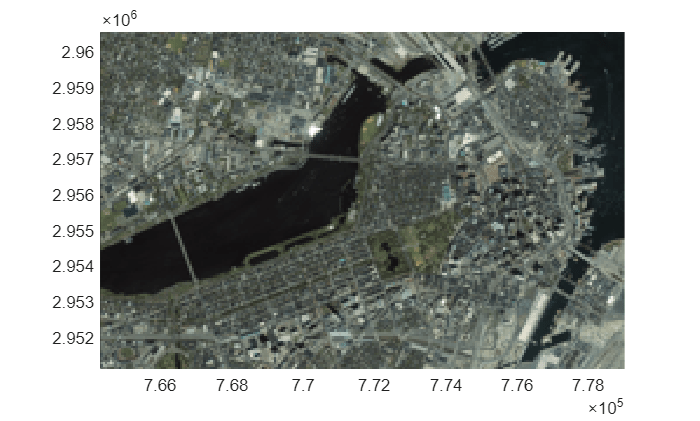Main Content

# mapresize

Resize projected raster

## Syntax

``[B,RB] = mapresize(A,RA,scale)``
``[B,RB] = mapresize(___,method)``
``[B,RB] = mapresize(___,'Antialiasing',TF)``

## Description

example

````[B,RB] = mapresize(A,RA,scale)` returns a raster `B` that is `scale` times the size of raster `A`. `RA` is a raster reference object that specifies the location and extent of data in `A`. `mapresize` returns the raster reference object `RB` that is associated with the returned raster `B`. By default, `mapresize` uses cubic interpolation.```
````[B,RB] = mapresize(___,method)` returns a resized raster where `method` specifies the interpolation method.```
````[B,RB] = mapresize(___,'Antialiasing',TF)` specifies whether to perform antialiasing when shrinking a raster. When `true`, `mapresize` performs antialiasing. The default value depends on the type of interpolation specified. For nearest-neighbor interpolation, the default value is `false`. For all other interpolation methods, the default is `true`.```

## Examples

collapse all

Import a sample projected raster and map cells reference object.

`[Z,R] = readgeoraster('map_sample.tif');`

Resize the raster using `mapresize`. Double the length and width of the raster by specifying the scale as 2. Use nearest neighbor interpolation by specifying the interpolation method as `'nearest'`.

`[Z2,R2] = mapresize(Z,R,2,'nearest');`

Verify the raster has been resized by comparing the size of the original raster with the size of the updated raster.

`R.RasterSize`
```ans = 1×2 2 2 ```
`R2.RasterSize`
```ans = 1×2 4 4 ```

If the rasters are small, you can compare them directly.

`Z`
```Z = 2×2 1 2 3 4 ```
`Z2`
```Z2 = 4×4 1 1 2 2 1 1 2 2 3 3 4 4 3 3 4 4 ```

Read a projected raster data set and map cells reference object into the workspace.

`[boston,R] = readgeoraster('boston.tif');`

Display the raster with `mapshow`.

`mapshow(boston,R)`Resize the projected raster data set. For this example, reduce the raster to one sixteenth of the original size.

`[resizedBoston,resizedR] = mapresize(boston,R,1/16);`

Display the resized raster. Note that `mapshow` preserves the original limits of the map in the display so that, at first glance, the resized raster appears to be the same size as the original. A closer look reveals that the size of pixels in the resized raster are larger than the pixels in the original.

```figure mapshow(resizedBoston,resizedR)```## Input Arguments

collapse all

Raster to be resized, specified as a numeric or logical array. If `A` has more than two dimensions, `mapresize` only resizes the first two dimensions.

Data Types: `single` | `double` | `int8` | `int16` | `int32` | `int64` | `uint8` | `uint16` | `uint32` | `uint64` | `logical`

Information about location and extent of raster, specified as a map raster reference object. To convert a raster matrix into a map raster reference object, use the `refmatToMapRasterReference` function.

Amount of resizing, specified as numeric scalar. If `scale` is in the range `[0 1]`, `B` is smaller than `A`. If `scale` is greater than 1, `B` is larger than `A`.

Example: `0.5`

Data Types: `single` | `double` | `int8` | `int16` | `int32` | `int64` | `uint8` | `uint16` | `uint32` | `uint64`

Interpolation method, specified as one of the following values.

ValueDescription
`'nearest'`Nearest-neighbor interpolation
`'bilinear'`Bilinear interpolation
`'cubic'`Cubic interpolation

Data Types: `char` | `string`

## Output Arguments

collapse all

Resized raster, returned as a numeric or logical array.

Information about location and extent of raster, returned as a map raster reference object.

## Tips

• Use `mapresize` with raster data in x- and y-coordinates. To work with geographic raster data in latitude and longitude coordinates, use `georesize`.

## See Also

Introduced in R2019a

## Support평가판 신청